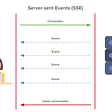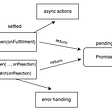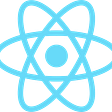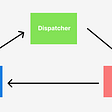# First steps in WebGL

Original post: https://aralroca.com/blog/first-steps-in-webgl

In this article, we’ll see what WebGL is and how to draw a triangle by talking to the graphics processing unit (GPU). Although this simple example could be solved in better ways, such as using a canvas with a 2d context or even with CSS, what we want is to start with WebGL. Like a “hello world”, to understand how it works.

We will cover the following:

# What is WebGL?

The literal definition of WebGL is “Web Graphics Library”. However, it is not a 3D library that offers us an easy-to-use API to say: «put a light here, a camera there, draw a character here, etc».

It’s in a low-level that converts vertices into pixels. We can understand WebGL as a rasterization engine. It’s based on OpenGL ES 3.0 graphical API (WebGL 2.0, unlike the old version that is based on ES 2.0).

The existing 3d libraries on the web (like THREE.js or Babylon.js) use WebGL below. They need a way to communicate to the GPU to tell what to draw.

This example could also be directly solved with THREE.js, using the `THREE.Triangle`. You can see an example here. However, the purpose of this tutorial is to understand how it works underneath, i.e. how these 3d libraries communicate with the GPU via WebGL. We are going to render a triangle without the help of any 3d library.

# Creating a WebGL canvas

In order to draw a triangle, we need to define the area where it is going to be rendered via WebGL.

We are going to use the element canvas of HTML5, retrieving the context as `webgl2`.

`import { useRef, useEffect } from 'preact/hooks'export default function Triangle() {  const canvas = useRef()  useEffect(() => {    const bgColor = [0.47, 0.7, 0.78, 1] // r,g,b,a as 0-1    const gl = canvas.current.getContext('webgl2') // WebGL 2.0    gl.clearColor(bgColor) // set canvas background color    gl.clear(gl.DEPTH_BUFFER_BIT | gl.COLOR_BUFFER_BIT) // clear buffers    // @todo: Render the triangle...  }, [])  return <canvas style={{ width: '100vw', height: '100vh' }} ref={canvas} />}`

The `clearColor` method sets the background color of the canvas using RGBA (with values from 0 to 1).

Furthermore, the `clear` method clears buffers to preset values. Used constants values are going to depend on your GPU capacity.

Once we have the canvas created, we are ready to render the inside triangle using WebGL… Let’s see how.

# Vertex coordinates

First of all, we need to know that all these vectors range from -1 to 1.

Corners of the canvas:

• (0, 0) — Center
• (1, 1) — Top right
• (1, -1) — Bottom right
• (-1, 1) — Top left
• (-1, -1) — Bottom left

The triangle we want to draw has these three points:

(-1, -1), (0, 1) and (1, -1). Thus, we are going to store the triangle coordinates into an array:

`const coordinates = [-1, -1, 0, 1, 1, -1]`

A shader is a type of computer program used in computer graphics to calculate rendering effects with high degree of flexibility. These shaders are coded and run on the GPU, written in OpenGL ES Shading Language (GLSL ES), a language similar to C or C++.

Each WebGL program that we are going to run is composed by two shader functions; the vertex shader and the fragment shader.

Almost all the WebGL API is made to run these two functions (vertex and fragment shaders) in different ways.

The job of the vertex shader is to compute the positions of the vertices. With this result (gl_Position) the GPU locates points, lines and triangles on the viewport.

To write the triangle, we are going to create this vertex shader:

`const vertexShader = `#version 300 es  precision mediump float;  in vec2 position;  void main () {      gl_Position = vec4(position.x, position.y, 0.0, 1.0); // x,y,z,w  }``

We can save it for now in our JavaScript code as a template string.

The first line (`#version 300 es`) tells the version of GLSL we are using.

The second line (`precision mediump float;`) determines how much precision the GPU uses to calculate floats. The available options are `highp`, `mediump` and `lowp`), however, some systems don't support `highp`.

In the third line (`in vec2 position;`) we define an input variable for the GPU of 2 dimensions (X, Y). Each vector of the triangle is in two dimensions.

The `main` function is called at program startup after initialization (like in C / C++). The GPU is going to run its content (`gl_Position = vec4(position.x, position.y, 0.0, 1.0);`) by saving to the `gl_Position` the position of the current vertex. The first and second argument are `x` and `y` from our `vec2` position. The third argument is the `z` axis, in this case is `0.0` because we are creating a geometry in 2D, not 3D. The last argument is `w`, by default this should be set to `1.0`.

The GLSL identifies and uses internally the value of `gl_Position`.

Once we create the shader, we should compile it:

`const vs = gl.createShader(gl.VERTEX_SHADER)gl.shaderSource(vs, vertexShader)gl.compileShader(vs)// Catch some possible errors on vertex shaderif (!gl.getShaderParameter(vs, gl.COMPILE_STATUS)) {  console.error(gl.getShaderInfoLog(vs))}`

After the “vertex shader”, the “fragment shader” is executed. The job of this shader is to compute the color of each pixel corresponding to each location.

For the triangle, let’s fill with the same color:

`const fragmentShader = `#version 300 es  precision mediump float;  out vec4 color;  void main () {      color = vec4(0.7, 0.89, 0.98, 1.0); // r,g,b,a  }`const fs = gl.createShader(gl.FRAGMENT_SHADER)gl.shaderSource(fs, fragmentShader)gl.compileShader(fs)// Catch some possible errors on fragment shaderif (!gl.getShaderParameter(fs, gl.COMPILE_STATUS)) {  console.error(gl.getShaderInfoLog(fs))}`

The syntax is very similar to the previous one, although the `vect4` we return here refers to the color of each pixel. Since we want to fill the triangle with `rgba(179, 229, 252, 1)`, we'll translate it by dividing each RGB number by 255.

Once we have the shaders compiled, we need to create the program that will run the GPU, adding both shaders.

`const program = gl.createProgram()gl.attachShader(program, vs) // Attatch vertex shadergl.attachShader(program, fs) // Attatch fragment shadergl.linkProgram(program) // Link both shaders togethergl.useProgram(program) // Use the created program// Catch some possible errors on programif (!gl.getProgramParameter(program, gl.LINK_STATUS)) {  console.error(gl.getProgramInfoLog(program))}`

# Create buffers

We are going to use a buffer to allocate memory to GPU, and bind this memory to a channel for CPU-GPU communications. We are going to use this channel to send our triangle coordinates to the GPU.

`// allowcate memory to gpuconst buffer = gl.createBuffer()// bind this memory to a channelgl.bindBuffer(gl.ARRAY_BUFFER, buffer)// use this channel to send data to the GPU (our triangle coordinates)gl.bufferData(  gl.ARRAY_BUFFER,  new Float32Array(coordinates),  // In our case is a static triangle, so it's better to tell  // how are we going to use the data so the WebGL can optimize  // certain things.  gl.STATIC_DRAW)// desallocate memory after send data to avoid memory leak issuesgl.bindBuffer(gl.ARRAY_BUFFER, null)`

# Link data from CPU to GPU

In our vertex shader, we defined an input variable named `position`. However, we haven't yet specified that this variable should take the value that we are passing through the buffer. We must indicate it in the following way:

`const position = gl.getAttribLocation(program, 'position')gl.enableVertexAttribArray(position)gl.bindBuffer(gl.ARRAY_BUFFER, buffer)gl.vertexAttribPointer(  position, // Location of the vertex attribute  2, // Dimension - 2D  gl.FLOAT, // Type of data we are going to send to GPU  gl.FALSE, // If data should be normalized  0, // Stride  0 // Offset)`

# Drawing the triangle

Once we have created the program with the shaders for our triangle and created the linked buffer to send data from the CPU to the GPU, we can finally tell the GPU to render the triangle!

`gl.drawArrays(  gl.TRIANGLES, // Type of primitive  0, // Start index in the array of vector points  3 // Number of indices to be rendered)`

This method renders primitives from array data. The primitives are points, lines or triangles. Let’s specify `gl.TRIANGLES`.

# All the code together

I’ve uploaded the article code to CodeSandbox in case you want to explore it.

# Conclusion

With WebGL, it is only possible to draw triangles, lines or points because it only rasterizes, so you can only do what the vectors can do. This means that WebGL is conceptually simple, while the process is quite complex… And gets more and more complex depending on what you want to develop. It’s not the same to rasterize a 2D triangle than a 3D videogame with textures, varyings, transformations…

I hope this article has been useful to understand a little bit of how WebGL works. I recommend a reading of the references below.

# References

--

--

--

## More from Aral Roca Gomez

Love podcasts or audiobooks? Learn on the go with our new app.

## Real-time data streaming to UI using Server-Sent Events for IoT applications## Promise? (JavaScript)## Using the Ethereum Web3 Library to Send Transactions in Moonbeam## Why Vuejs Is Considered As the Best Front End Development Framework Today?## My React Native Journey — part 1: Introduction, Resources## [Frontend] One-way binding vs Two-way binding## Tailwind The Early Lessons: Setup Test Environment In Under 60 Seconds## Choosing a new web stack.